TitleIntermediate Algebra
Answer/Discussion to Practice Problems
Tutorial 3: Sets of NumbersAnswer/Discussion to 1a {x | x is a natural number less than 4}   There are two parts to this: the number has to belong to the set of natural numbers {1, 2, 3, 4, ...}  and  it has to be less than 4. Putting these two ideas together we get {1, 2, 3} (return to problem 1a)Answer/Discussion to 1b  {x | x is a whole number between 100 and 105}   There are two parts to this: the number has to belong to the set of whole numbers {0, 1, 2, 3, 4, ...}  and  it has to be between 100 and 105, not including 100 and 105. Putting these two ideas together we get {101, 102, 103, 104} (return to problem 1b)Answer/Discussion to 1c {x | x is an odd whole number greater than 5}   There are three parts to this: the number has to belong to the set of whole numbers {0, 1, 2, 3, 4, ...}  and  it has to be greater than 5 and it has to be odd. Putting these two ideas together we get {7, 9, 11, 13 ...} (return to problem 1c)Answer/Discussion to 2a {-3, -3/2, 0, .25, 1} Note that we have two non-integer numbers to graph.  The -3/2 will go halfway between -2 and -1.  The .25 will go between 0 and 1, a little closer to 0 than 1.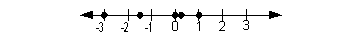(return to problem 2a)Answer/Discussion to 3a Natural numbers The numbers in the given set that are also natural numbers are {2,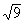}. Note thatsimplifies to be 3 which is a natural number. (return to problem 3a)Answer/Discussion to 3b Whole numbers The numbers in the given set that are also whole numbers are {0, 2,}. (return to problem 3b)Answer/Discussion to 3c Integers The numbers in the given set that are also integers are {0, 2,}. (return to problem 3c)Answer/Discussion to 3d Rational numbers The numbers in the given set that are also rational numbers are {-1.5, 0, 2,}. (return to problem 3d)Answer/Discussion to 3e Irrational numbers The number in the given set that is also an irrational number is {}. (return to problem 3e)Answer/Discussion to 3f Real numbers The numbers in the given set that are also real numbers are {-1.5, 0, 2,,}. (return to problem 3f)Answer/Discussion to 4a  0  ? {x | x is a rational number} Since 0 can be written as 0/1 (one integer over another), it is a part of the list of rational numbers.  So it would be true to say 0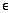{x | x is a rational number}. (return to problem 4a)Answer/Discussion to 4b 10  ? { 2, 4, 6, 8, ...} The ellipsis in the set indicate that the pattern of numbers (even natural numbers) would continue, which means 10 would be included in this list.  So it would be true to say 10{2, 4, 6, 8, ...}. (return to problem 4b)Answer/Discussion to 4c?  {x | x is an irrational number} Sincesimplifies to be 2, which is a rational number, it can not be an irrational number.  Therefore,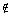{x | x is an irrational number}. (return to problem 4c)Answer/Discussion to 5a Q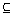R Since EVERY element of Q (rational numbers) is also in R (Real numbers),  this statement is TRUE. (return to problem 5a)Answer/Discussion to 5b RZ Since EVERY element of R (Real numbers) is not also in Z (Integers),  this statement is FALSE. (return to problem 5b)Answer/Discussion to 6a  |10| When looking for the absolute value of 10, we are looking for the number of units (or distance) 10 is from 0 on the number line. I came up with 10, how about you? (return to problem 6a)Answer/Discussion to 6b - |-10|  This problem has a little twist to it.  Let's talk it through.  First of all, if we just concentrate on  |-10| we would get 10.  Then, note that there is a negative on the OUTSIDE of the absolute value.  That means we are going to negate what we get for the absolute value.  Putting that together we get -10 for our answer.   Note that the absolute value part of the problem was still positive.  We just had a negative on the outside of it that made the final answer negative. (return to problem 6b)Answer/Discussion to 7a ½ The opposite of 1/2 is -1/2, since both of these numbers have the same absolute value but are on opposite sides of the origin on the number line. (return to problem 7a)Answer/Discussion to 7b -20 The opposite of -20 is 20, since both of these numbers have the same absolute value but are on opposite sides of the origin on the number line. (return to problem 7b)

Last revised on June 10, 2011 by Kim Seward.
All contents copyright (C) 2002 - 2011, WTAMU and Kim Seward. All rights reserved.